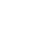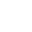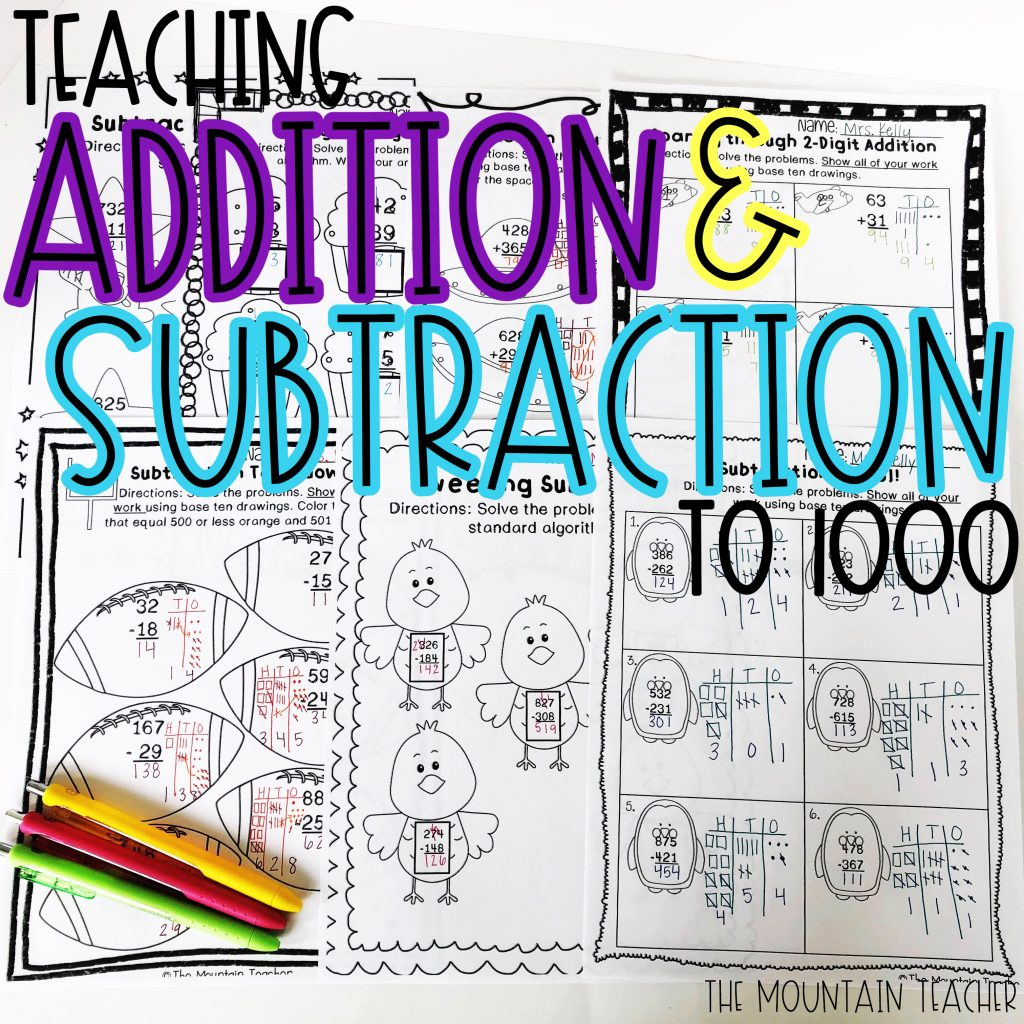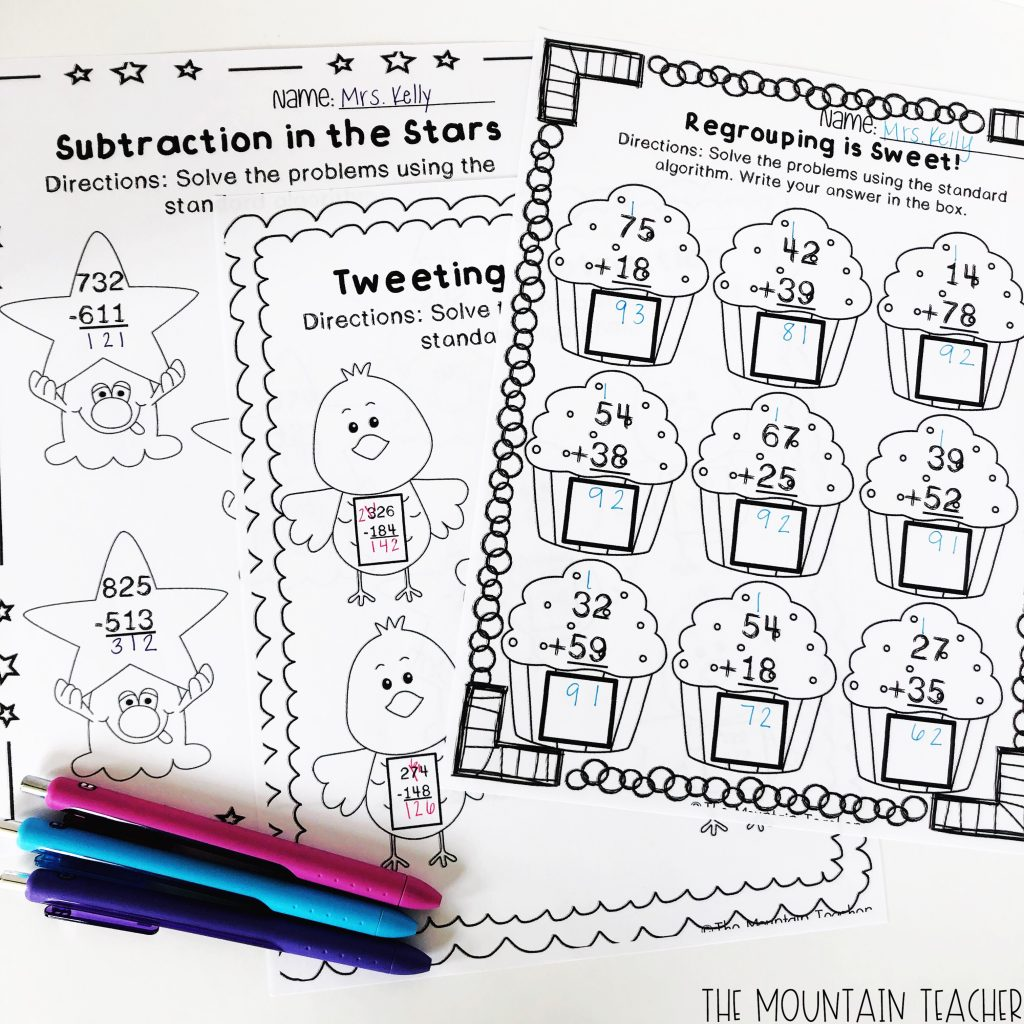# Addition and Subtraction to 1000

If you teach second grade, then you know that one of the most dreaded math standards out there is CCSS 2 NBT B 7…. adding and subtracting up to 1000 using concrete models or strategies based on place value. While there are many ways to attack this standard, I have found much success teaching this using the following protocol.#### Place Value Foundation

You NEED to make sure your students have a solid understanding of place value before even attempting to teach this unit. Students need to be able to represent all numbers up to 1000 using base ten representations. They also need to understand that ten ones makes a ten stick, that ten tens sticks make a one-hundred and that ten hundreds make one one-thousand cube. Students usually understand this fairly quickly, and this is a unit we teach for about two weeks right at the beginning of the year.

#### Move Slow to Go Fast

We usually teach this addition and subtraction unit for SIX weeks. Yes, you read that correctly. It takes us a minimum of six weeks to truly be sure students not only understand how to add and subtract fluently, but understand the process behind the equations that they are eventually able to plug and chug.

So what do we do in all that time? I spend the first week teaching students how to add and subtract 2/3 digit numbers that do not involve regrouping. I will always start using a model of two different colored base ten blocks, but quickly move away from the actual manipulatives and use drawings as this is a much faster, much more efficient way for students to learn to add and subtract.

The next four weeks are all spent adding and subtracting using base ten models. I usually introduce the kids to two different models: number lines and base ten drawings. The students usually prefer the drawings because they are more concrete. Finally, we spend the last two weeks learning and practicing the standard algorithm. I spend one week on adding and one on subtracting. I would highly recommend teaching these in concrete isolation before mixing them together to avoid confusion on regrouping.

#### Adding and Subtracting Without Regrouping using Base Ten

For some students, it might help for them to draw each number in a different color to start. Then, I just make sure the students are making their drawings organized. We do this by using a base ten chart. We repeat many, many, many times a day that “we always start with our ones” and we practice this. We do this when we draw our picture as well as when we go to add the numbers. This just helps students remember and be consistent. You can see an example of how we add and subtract below.#### Adding and Subtracting With Regrouping using Base Ten

When students get to the counting step of adding or run out of numbers when they are subtracting, we always shout out “regroup” when we get to ten, or if we need more. This is an auditory reminder of exactly WHAT is happening when you run into that situation. We ALWAYS talk about what this means. Every single day when we are working whole group, I tell students to turn and tell their neighbor what regrouping means. We go over it again and again and again. We also talk again and again and again about what the difference in regrouping for addition and subtraction is. Repetition is truly key in having students remember this essential skill, as is being able to see the base ten representations.#### Adding and Subtracting Using the Standard Algorithm

When it comes time to teach the standard algorithm, I always show them the problem using a base ten drawing first. Then, we re-do the problem using the standard algorithm. We talk about how it is so important to start with our ones still, and talk about why. Then, we practice different ways to add the numbers quickly. Students usually “grab” onto the top number and either count forward or backward the second number. Struggling students will benefit greatly from having a number line available. We talk about if the answer in addition is 14, the ten goes above the tens, and the ones get brought under the ones. I also make sure students know the “1” being added is actually a group of 10 or 100. When we do subtraction, we always repeat the poem “More on top? No need to stop. More on the floor? Go next door and get ten more.” This always helps students remember when to regroup and not to regroup. I find that students need much more time practicing subtraction with regrouping than addition with regrouping, and I always tell students they can use either method to constantly be checking that their answers are correct.#### Other Tips

• If students already have been taught at home or somewhere else how to add/subtract using the standard algorithm, I always allow them to use that method to check their work. I tell them the importance of knowing it both ways, and ensure them that they will be experts once we get there.
• I would highly recommend not mixing the two strategies together. This will create confusion with regrouping. I strongly recommend teaching the base ten models first, and once they are ALL experts at it, then you can introduce the standard algorithm. If students do not understand the base ten models, they will not understand the mathematics that are behind adding and subtracting multi-digit numbers. If they are taught the standard algorithm before having a solid understanding of the pictures, they often rely too heavily on that and never learn both ways. Understanding this will help them understand the different between addition, subtraction, multiplication and division down the road.
• I cannot emphasize enough how important it is that students have a solid understanding of place value before learning how to add and subtract. If they do not know this, they will not be able to draw and fully understand the place value of each number.
• Math facts are super important for young learners, especially when it comes to the standard algorithm. Students will be able to complete problems much faster if they are able to do the small math in their heads.
• If students do not show their work on regrouping, whether it is using base ten models or crossing out/adding numbers at the top, I mark their work wrong. Typically, we discourage students trying to add and subtract with regrouping in their head as this can lead to small errors that are hard to correct if you cannot see what the error is.
• Creating anchor charts with your class as a visual for students to refer back to is a great way to foster more independence. I detail each step that we do, and try to have between 3-5 steps for each different strategy we learn how to do. This is a great reference for students when they are trying something new for the first time, but it is also encouraged to eventually remove this crutch so students can start working through the problems more individually as well.

#### Available Resources

Do you teach this with your class? What questions or wonderings do you still have? Drop them or any suggestions for others in the comments below! Thanks!